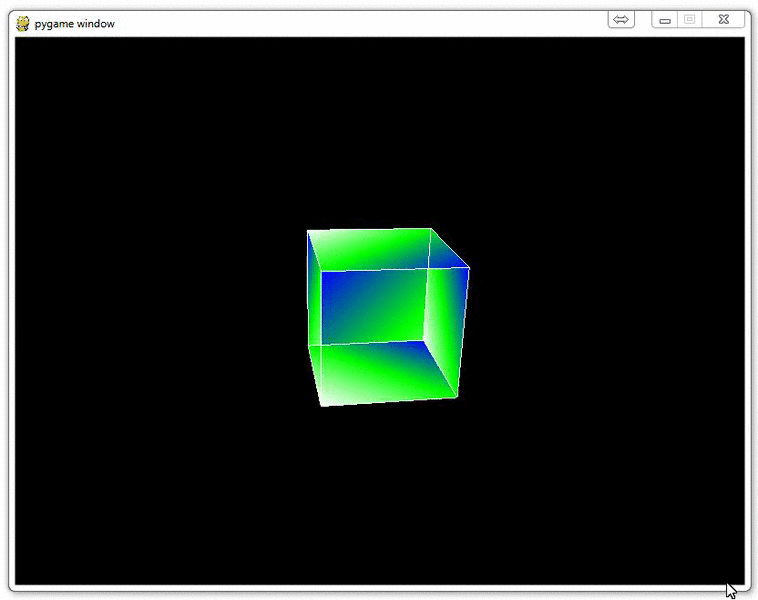## Understanding navigation within the 3D environment via OpenGL

Now that you have drawn a 3D object, and shown it moving, it's time to show why a real 3D environment really shines: Navigating the environment yourself.

This can come in two major ways, but can be quite confusing. The question you need to answer at the end of this tutorial is: Are you moving the object, or are you moving your perspective of the object? So, in the previous tutorial, are we spinning the cube, or are we rotating around the cube? In this tutorial, are we moving the cube, or are we moving around the cube?

So let's say our objective is to "move the cube" with our arrow keys. How might we do this?

Now, we make our main function look like:

```def main():
pygame.init()
display = (800,600)
pygame.display.set_mode(display, DOUBLEBUF|OPENGL)

gluPerspective(45, (display/display), 0.1, 50.0)

glTranslatef(0,0, -10)

glRotatef(25, 2, 1, 0)

while True:
for event in pygame.event.get():
if event.type == pygame.QUIT:
pygame.quit()
quit()

if event.type == pygame.KEYDOWN:
if event.key == pygame.K_LEFT:
glTranslatef(-0.5,0,0)
if event.key == pygame.K_RIGHT:
glTranslatef(0.5,0,0)

if event.key == pygame.K_UP:
glTranslatef(0,1,0)
if event.key == pygame.K_DOWN:
glTranslatef(0,-1,0)

if event.type == pygame.MOUSEBUTTONDOWN:
if event.button == 4:
glTranslatef(0,0,1.0)

if event.button == 5:
glTranslatef(0,0,-1.0)

#glRotatef(1, 3, 1, 1)
glClear(GL_COLOR_BUFFER_BIT|GL_DEPTH_BUFFER_BIT)
Cube()
pygame.display.flip()
pygame.time.wait(10)
```

Notice the main change being the keydown and keyup events. Also, note the pygame.MOUSEBUTTONDOWN. pygame.MOUSEBUTTONDOWN allows us to pull data from the user's mouse wheel, so we can have it perform an expected action.

Now, again, is it the cube that is moving, or is it our perspective? Think about X, Y, Z, what direction we're "moving" whatever is being moved, and what we're seeing as a result.The next tutorial:• OpenGL with PyOpenGL introduction and creation of Rotating Cube

• Coloring Surfaces as well as understand some of the basic OpenGL code

• Understanding navigation within the 3D environment via OpenGL
• Moving the player automatically towards the cube

• Random Cube Position

• Adding Many Cubes to our Game

• Adding a ground in OpenGL

• Infinite flying cubes

• Optimizing the processing for infinite cubes